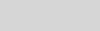# Introducing Tensor for multi-dimensional Machine Learning and AI data.NET Team

## Overview

Tensor is an exchange type for homogenous multi-dimensional data for 1 to N dimensions. The motivation behind introducing Tensor<T> is to make it easy for Machine Learning library vendors like CNTK, Tensorflow, Caffe, Scikit-Learn to port their libraries over to .NET with minimal dependencies in place.  Tensor<T> is designed to provide the following characteristics.

• Good exchange type for multi-dimensional machine-learning data
• Support for different sparse and dense layouts
• Efficient interop with native Machine Learning Libraries using zero copies
• Work with any type of memory (Unmanaged, Managed)
• Allow for slicing and indexing Machine Learning data efficiently
• Basic math and manipulation
• Fits existing API patterns in the .NET framework like Vector<T>
• Supports for .NET Framework, .NET Core, Mono, Xamarin & UWP
• Doesn’t replace existing Math or Machine Learning libraries. Instead the goal is to give those libraries a sufficient exchange type for multi-dimensional data to avoid reimplementing, copying data, or depending on one another.

If you familiar with recent work with Memory<T>, Tensor<T> can be thought of as extending Memory<T> for multiple dimensions and sparse data. Primary attributes for defining a Tensor<T> include:

• Type T: Primitive (e.g. int, float, string…) and non-primitive types
• Dimensions: The shape of the Tensor (e.g. 3×5 matrix, 28x28x3, etc.)
• Layout (Optional): Row vs. Column major. The API calls this “ReverseStride” to generalize to many dimensions instead of just “row” and “column”. The default is reverseStride: false (i.e. row-major).
• Memory (Optional): The backing storage for the values in the Tensor, which is used in-place without copying.

You can try out Tensor<T> with CNTK for the Pre-built MNIST model by following this GitHub repo.

## Types of Tensor

There are currently three tensor types that we support as a part of this work. The following table summarizes Dense, Sparse and CompressedSparse Tensor along with providing performance characteristics and recommended usage patterns for each one of them.

 Dense Tensor Compressed Sparse Tensor Sparse Tensor Description Stores values in a contiguous sequential block of memory where all values are represented. A tensor that where only the non-zero values are represented. For a two-dimensional tensor this is referred to as compressed sparse row (CSR, CRS, Yale), compressed sparse column (CSC, CCS). CompressedSparseTensor is great for interop and serialization but bad for mutation due to reallocation and data shifting. A tensor that where only the non-zero values are represented. SparseTensor’s backing storage is a Dictionary where the key is the linearized index of the n-dimension indices. SparseTensor is meant as an intermediate to be used to build other Tensors, such as CompressedSparseTensor. Unlike CompressedSparseTensor where insertions are O(n), insertions to SparseTensor are nominally O(1). Create O(n) O(capacity) O(capacity) Memory O(n) O(nnz) O(nnz) Access O(1) O(log nonCompressedDimensions) O(1) Insert/Remove N/A O(nnz) O(1) Similarity numpy.ndarray sparse.csr_matrix/ sparse.csc_matrix in scipy in scipy sparse.dok_matrix in scipy Example  (C#) var denseTensor = new DenseTensor(new[] { 3, 5 }); var compressedSparseTensor = new CompressedSparseTensor(new[] { 3, 5 }); var sparseTensor = new SparseTensor(new[] { 3, 5 });

One of the additional advantages of using Tensor is that Tensor would do the offset calculation (stride) for you based upon the shape and the dimensions specified during Tensor allocation, this will save you the added complexity that comes with doing the offset calculation yourself. The example below, illustrates this in detail.## Current Limitations & Non-goals

• The current arithmetic operations are rudimentary and bare essentials at this point.
• There hasn’t been any optimization effort applied. We are considering if these are necessary for a “v1” of Tensor or if they can be trimmed from the initial release.
• With Tensor<T> we are not looking to build a canonical linear-algebra library or machine learning implementation into .NET.
• One of the other non-goals with Tensor<T> is build device-specific memory allocation and arithmetic into Tensor e.g. GPU, FPGA. We imagine these scenarios can be implemented in a library outside of the Tensor library.

## Future Work

• Potentially growing the suite of manipulations and arithmetic to be a good, stable, useful set.
• Improve performance of these methods using vectorization, and specialized CPU instructions like SIMD.
• Grow the set of implementations of tensor to represent all common layouts
• Continue working on open issues on our  Tensor<T> github repo.